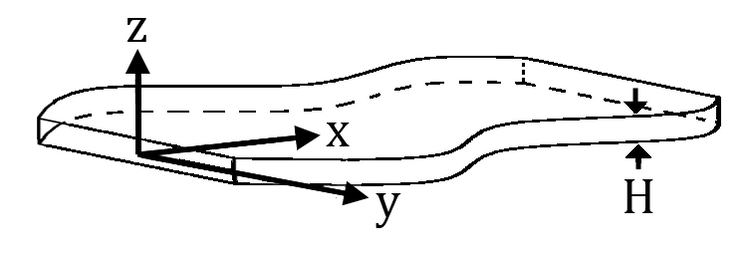# Hele Shaw flow

Updated onHele-Shaw flow (named after Henry Selby Hele-Shaw) is defined as Stokes flow between two parallel flat plates separated by an infinitesimally small gap. Various problems in fluid mechanics can be approximated to Hele-Shaw flows and thus the research of these flows is of importance. Approximation to Hele-Shaw flow is specifically important to micro-flows. This is due to manufacturing techniques, which creates shallow planar configurations, and the typically low Reynolds numbers of micro-flows.

## Contents

The governing equation of Hele-Shaw flows is identical to that of the inviscid potential flow and to the flow of fluid through a porous medium (Darcy's law). It thus permits visualization of this kind of flow in two dimensions.

## Mathematical formulation of Hele-Shaw flows

Let x , y be the directions parallel to the flat plates, and z the perpendicular direction, with 2 H being the gap between the plates (at z = ± H ). When the gap between plates is asymptotically small

H 0 ,

the velocity profile in the z direction is parabolic (i.e. is a quadratic function of the coordinate in this direction). The equation relating the pressure gradient to the velocity is,

u = p z 2 H 2 2 μ

where u is the velocity , p ( x , y , t ) is the local pressure, μ is the fluid viscosity.

This relation and the uniformity of the pressure in the narrow direction z permits us to integrate the velocity with regard to z and thus to consider an effective velocity field in only the two dimensions x and y . When substituting this equation into the continuity equation and integrating over z we obtain the governing equation of Hele-Shaw flows,

2 p x 2 + 2 p y 2 = 0.

This equation is supplemented by the no-penetration boundary conditions on the side walls of the geometry,

p n ^ = 0

where n ^ is a unit vector perpendicular to the side wall.

## Hele-Shaw cell

The term Hele-Shaw cell is commonly used for cases in which a fluid is injected into the shallow geometry from above or below the geometry, and when the fluid is bounded by another liquid or gas. For such flows the boundary conditions are defined by pressures and surface tensions.

## References

Similar Topics
Four Jacks and a Jill (film)
Eyal Maoz
Fala Chen
Topics